# Introduction to Programming in MATLAB Intro MATLAB Peer

• Slides: 8
Download presentation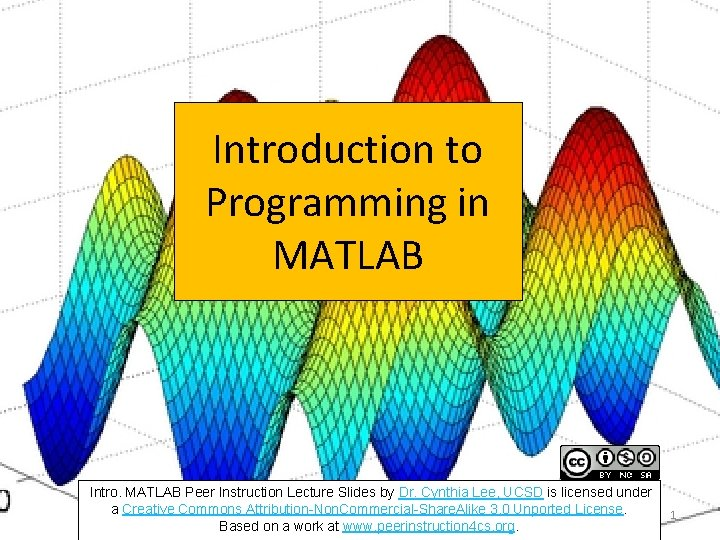Introduction to Programming in MATLAB Intro. MATLAB Peer Instruction Lecture Slides by Dr. Cynthia Lee, UCSD is licensed under a Creative Commons Attribution-Non. Commercial-Share. Alike 3. 0 Unported License. Based on a work at www. peerinstruction 4 cs. org. 1Making a Sunset >> im(: , 2) = im(: , 2) *. 6; >> im(: , 3) = im(: , 3) *. 6; 2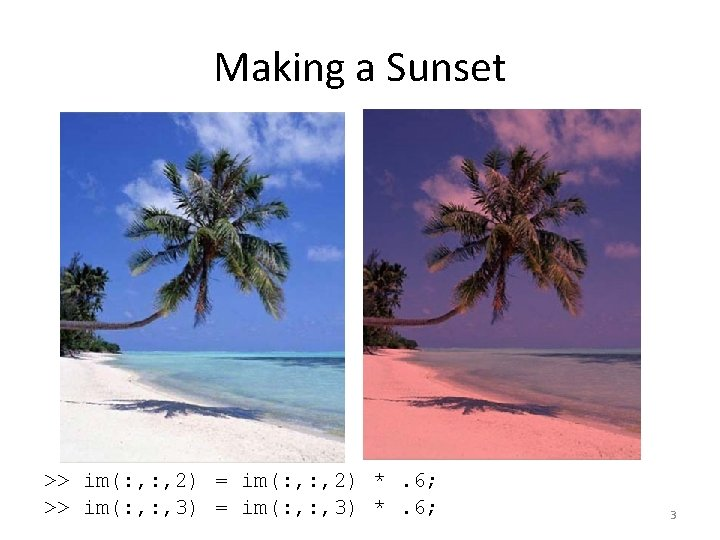Making a Sunset >> im(: , 2) = im(: , 2) *. 6; >> im(: , 3) = im(: , 3) *. 6; 3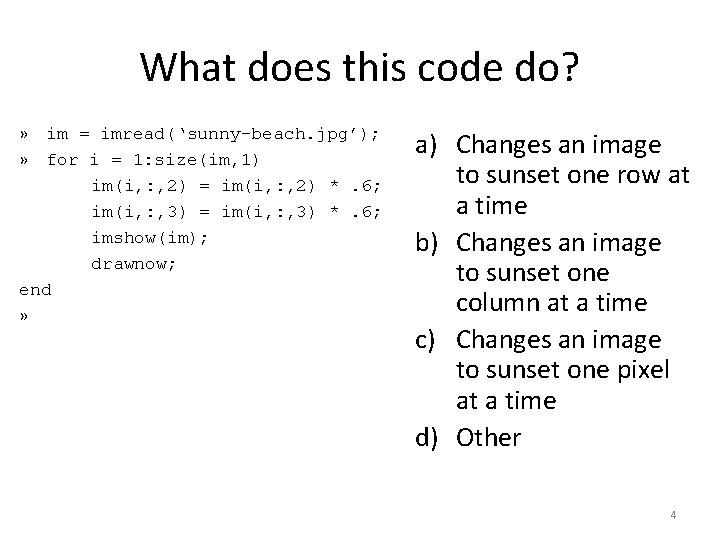What does this code do? » im = imread(‘sunny-beach. jpg’); » for i = 1: size(im, 1) im(i, : , 2) = im(i, : , 2) *. 6; im(i, : , 3) = im(i, : , 3) *. 6; imshow(im); drawnow; end » a) Changes an image to sunset one row at a time b) Changes an image to sunset one column at a time c) Changes an image to sunset one pixel at a time d) Other 4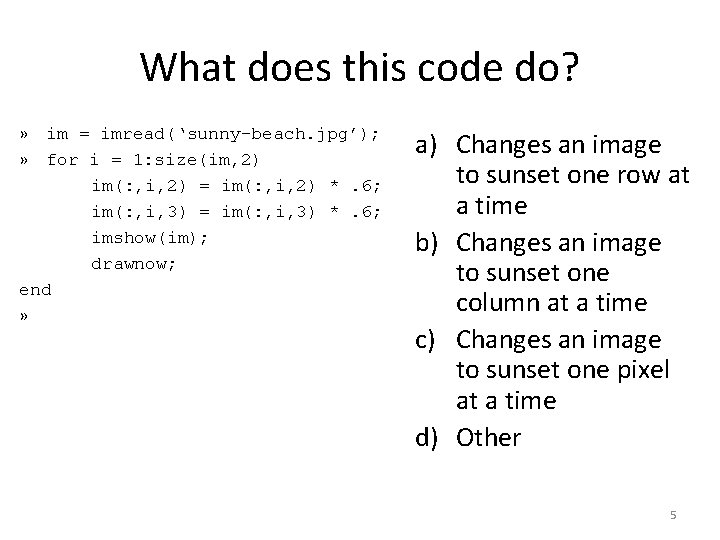What does this code do? » im = imread(‘sunny-beach. jpg’); » for i = 1: size(im, 2) im(: , i, 2) = im(: , i, 2) *. 6; im(: , i, 3) = im(: , i, 3) *. 6; imshow(im); drawnow; end » a) Changes an image to sunset one row at a time b) Changes an image to sunset one column at a time c) Changes an image to sunset one pixel at a time d) Other 5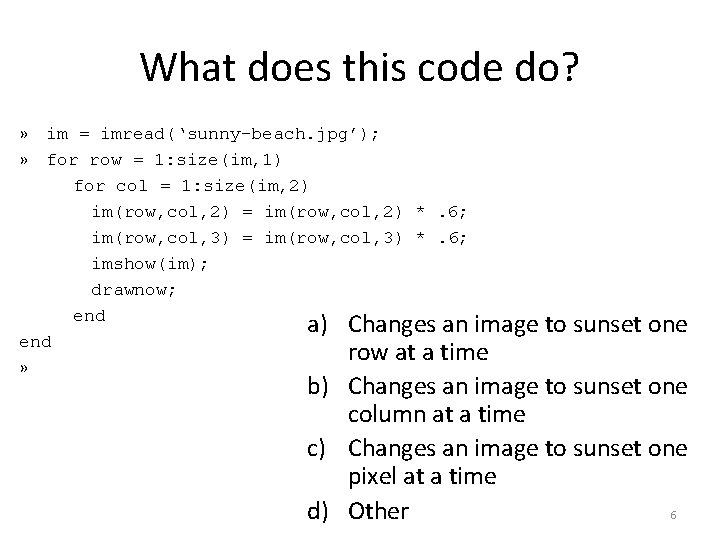What does this code do? » im = imread(‘sunny-beach. jpg’); » for row = 1: size(im, 1) for col = 1: size(im, 2) im(row, col, 2) = im(row, col, 2) *. 6; im(row, col, 3) = im(row, col, 3) *. 6; imshow(im); drawnow; end a) Changes an image end row at a time » to sunset one b) Changes an image to sunset one column at a time c) Changes an image to sunset one pixel at a time d) Other 6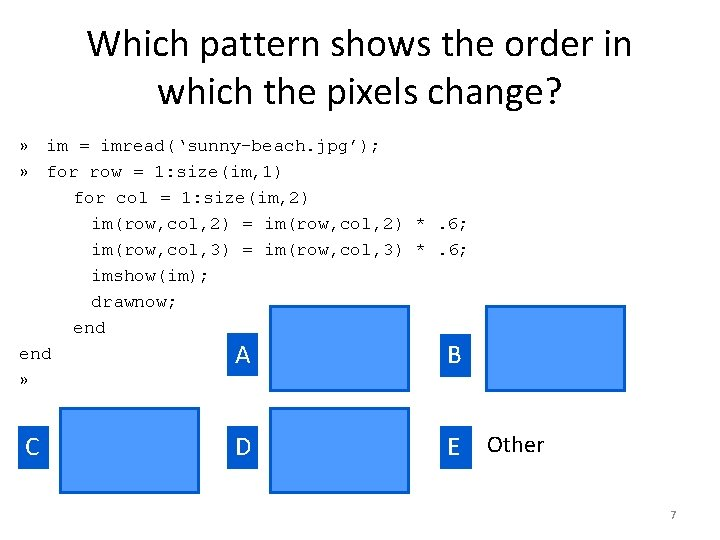Which pattern shows the order in which the pixels change? » im = imread(‘sunny-beach. jpg’); » for row = 1: size(im, 1) for col = 1: size(im, 2) im(row, col, 2) = im(row, col, 2) *. 6; im(row, col, 3) = im(row, col, 3) *. 6; imshow(im); drawnow; end A B » C D E Other 7Which code makes the pixels change this other way? » for row = 1: size(im, 1) Original for col = 1: size(im, 2) im(row, col, 2) = im(row, col, 2) *. 6; im(row, col, 3) = im(row, col, 3) *. 6; imshow(im); drawnow; end » for row = 1: size(im, 1) A for col = 1: size(im, 2) im(col, row, 2) = im(col, row, 2) *. 6; im(col, row, 3) = im(col, row, 3) *. 6; imshow(im); » for col = 1: size(im, 2) B drawnow; for row = 1: size(im, 1) end im(row, col, 2) = im(row, col, 2) *. 6; end im(row, col, 3) = im(row, col, 3) *. 6; imshow(im); Other, or can’t be drawnow; done end 8 end C